Project Description

In this project, you will learn to implement a trading strategy on your own, and test to see if it has the potential to be profitable. You will be supplied with a universe of stocks and time range. You will also be provided with a textual description of how to generate a trading signal based on a momentum indicator. You will then compute the signal for the time range given and apply it to the dataset to produce projected returns. Finally, you will perform a statistical test on the mean of the returns to conclude if there is alpha in the signal. For the dataset, we'll be using the end of day from Quotemedia.

Instructions

Each problem consists of a function to implement and instructions on how to implement the function. The parts of the function that need to be implemented are marked with a # TODO comment. After implementing the function, run the cell to test it against the unit tests we've provided. For each problem, we provide one or more unit tests from our project_tests package. These unit tests won't tell you if your answer is correct, but will warn you of any major errors. Your code will be checked for the correct solution when you submit it to Udacity.

Packages

When you implement the functions, you'll only need to you use the packages you've used in the classroom, like Pandas and Numpy. These packages will be imported for you. We recommend you don't add any import statements, otherwise the grader might not be able to run your code.

The other packages that we're importing are helper, project_helper, and project_tests. These are custom packages built to help you solve the problems. The helper and project_helper module contains utility functions and graph functions. The project_tests contains the unit tests for all the problems.

Install Packages

import sys
!{sys.executable} -m pip install -r requirements.txt
Requirement already satisfied: colour==0.1.5 in /opt/conda/lib/python3.6/site-packages (from -r requirements.txt (line 1))
Collecting cvxpy==1.0.3 (from -r requirements.txt (line 2))
[K    100% |████████████████████████████████| 880kB 520kB/s eta 0:00:01
[?25hRequirement already satisfied: cycler==0.10.0 in /opt/conda/lib/python3.6/site-packages/cycler-0.10.0-py3.6.egg (from -r requirements.txt (line 3))
Collecting numpy==1.13.3 (from -r requirements.txt (line 4))

You should consider upgrading via the 'pip install --upgrade pip' command.

import pandas as pd
import numpy as np
import helper
import project_helper
import project_tests

Market Data

The data we use for most of the projects is end of day data. This contains data for many stocks, but we'll be looking at stocks in the S&P 500. We also made things a little easier to run by narrowing down our range of time period instead of using all of the data.

df = pd.read_csv('../../data/project_1/eod-quotemedia.csv', parse_dates=['date'], index_col=False)

#print(close)
print('Loaded Data')
Loaded Data

View Data

Run the cell below to see what the data looks like for close.

project_helper.print_dataframe(close)Stock Example

Let's see what a single stock looks like from the closing prices. For this example and future display examples in this project, we'll use Apple's stock (AAPL). If we tried to graph all the stocks, it would be too much information.

apple_ticker = 'AAPL'
project_helper.plot_stock(close[apple_ticker], '{} Stock'.format(apple_ticker))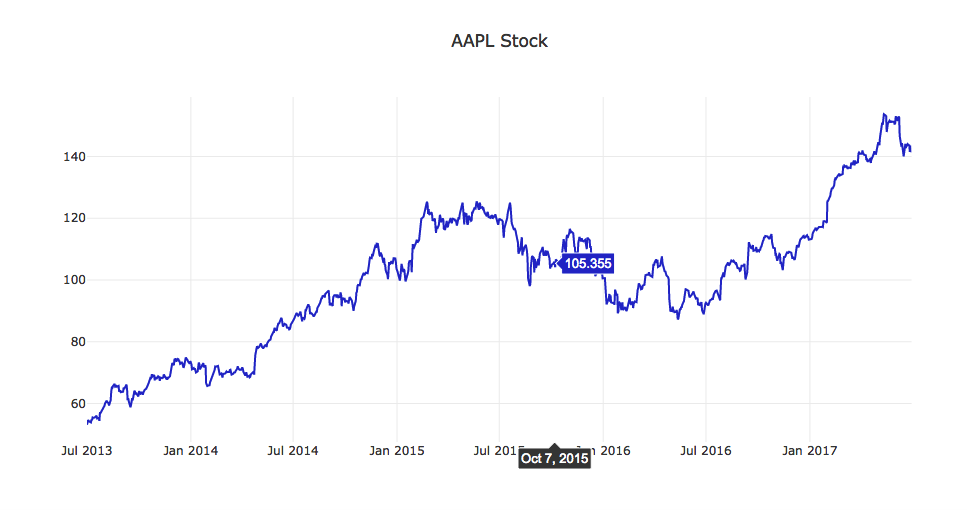The trading signal you'll develop in this project does not need to be based on daily prices, for instance, you can use month-end prices to perform trading once a month. To do this, you must first resample the daily adjusted closing prices into monthly buckets, and select the last observation of each month.

Implement the resample_prices to resample close_prices at the sampling frequency of freq.

def resample_prices(close_prices, freq='M'):
"""
Resample close prices for each ticker at specified frequency.

Parameters
----------
close_prices : DataFrame
Close prices for each ticker and date
freq : str
What frequency to sample at
For valid freq choices, see http://pandas.pydata.org/pandas-docs/stable/timeseries.html#offset-aliases

Returns
-------
prices_resampled : DataFrame
Resampled prices for each ticker and date
"""
# TODO: Implement Function
res = close_prices.groupby(pd.Grouper(freq=freq)).last()

# print(res)

return res

project_tests.test_resample_prices(resample_prices)
Tests Passed

View Data

Let's apply this function to close and view the results.

monthly_close = resample_prices(close)
project_helper.plot_resampled_prices(
monthly_close.loc[:, apple_ticker],
close.loc[:, apple_ticker],
'{} Stock - Close Vs Monthly Close'.format(apple_ticker))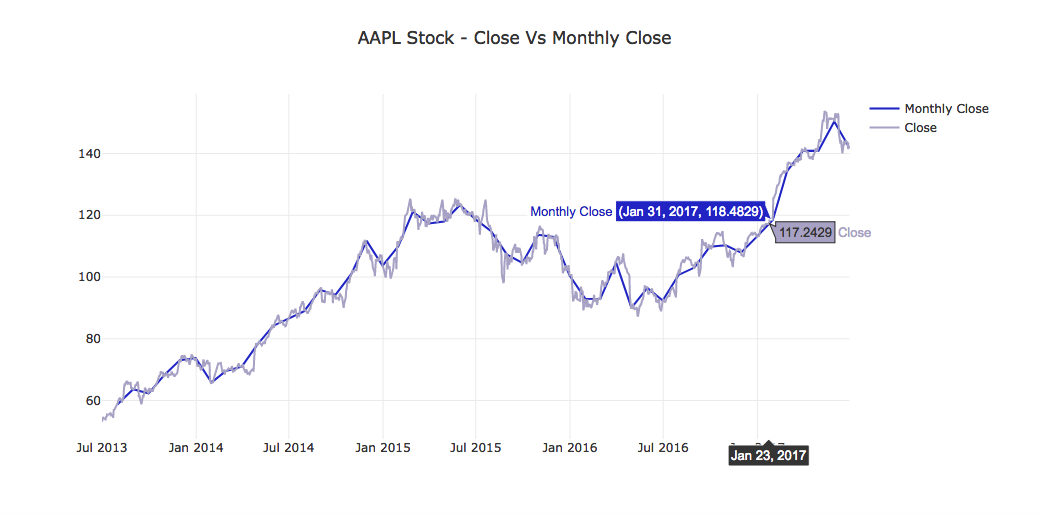Compute Log Returns

Compute log returns ($R_t$) from prices ($P_t$) as your primary momentum indicator:

$$R_t = log_e(P_t) - loge(P{t-1})$$

Implement the compute_log_returns function below, such that it accepts a dataframe (like one returned by resample_prices), and produces a similar dataframe of log returns. Use Numpy's log function to help you calculate the log returns.

def compute_log_returns(prices):
"""
Compute log returns for each ticker.

Parameters
----------
prices : DataFrame
Prices for each ticker and date

Returns
-------
log_returns : DataFrame
Log returns for each ticker and date
"""
# TODO: Implement Function

return np.log(prices) - np.log(prices.shift(1))

project_tests.test_compute_log_returns(compute_log_returns)
Tests Passed

View Data

Using the same data returned from resample_prices, we'll generate the log returns.

monthly_close_returns = compute_log_returns(monthly_close)
project_helper.plot_returns(
monthly_close_returns.loc[:, apple_ticker],
'Log Returns of {} Stock (Monthly)'.format(apple_ticker))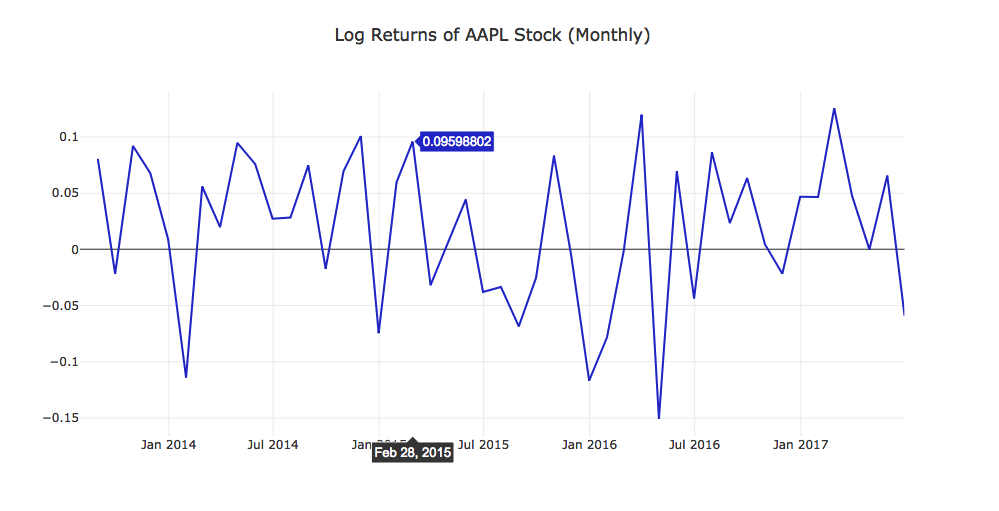Shift Returns

Implement the shift_returns function to shift the log returns to the previous or future returns in the time series. For example, the parameter shift_n is 2 and returns is the following:

                           Returns
A         B         C         D
2013-07-08     0.015     0.082     0.096     0.020     ...
2013-07-09     0.037     0.095     0.027     0.063     ...
2013-07-10     0.094     0.001     0.093     0.019     ...
2013-07-11     0.092     0.057     0.069     0.087     ...
...            ...       ...       ...       ...

the output of the shift_returns function would be:

                        Shift Returns
A         B         C         D
2013-07-08     NaN       NaN       NaN       NaN       ...
2013-07-09     NaN       NaN       NaN       NaN       ...
2013-07-10     0.015     0.082     0.096     0.020     ...
2013-07-11     0.037     0.095     0.027     0.063     ...
...            ...       ...       ...       ...

Using the same returns data as above, the shift_returns function should generate the following with shift_n as -2:

                        Shift Returns
A         B         C         D
2013-07-08     0.094     0.001     0.093     0.019     ...
2013-07-09     0.092     0.057     0.069     0.087     ...
...            ...       ...       ...       ...       ...
...            ...       ...       ...       ...       ...
...            NaN       NaN       NaN       NaN       ...
...            NaN       NaN       NaN       NaN       ...

Note: The "..." represents data points we're not showing.

def shift_returns(returns, shift_n):
"""
Generate shifted returns

Parameters
----------
returns : DataFrame
Returns for each ticker and date
shift_n : int
Number of periods to move, can be positive or negative

Returns
-------
shifted_returns : DataFrame
Shifted returns for each ticker and date
"""
# TODO: Implement Function
# print res
res = returns.shift(shift_n)
# print(res)

return res

project_tests.test_shift_returns(shift_returns)
Tests Passed

View Data

Let's get the previous month's and next month's returns.

prev_returns = shift_returns(monthly_close_returns, 1)

project_helper.plot_shifted_returns(
prev_returns.loc[:, apple_ticker],
monthly_close_returns.loc[:, apple_ticker],
'Previous Returns of {} Stock'.format(apple_ticker))
project_helper.plot_shifted_returns(
monthly_close_returns.loc[:, apple_ticker],
'Lookahead Returns of {} Stock'.format(apple_ticker))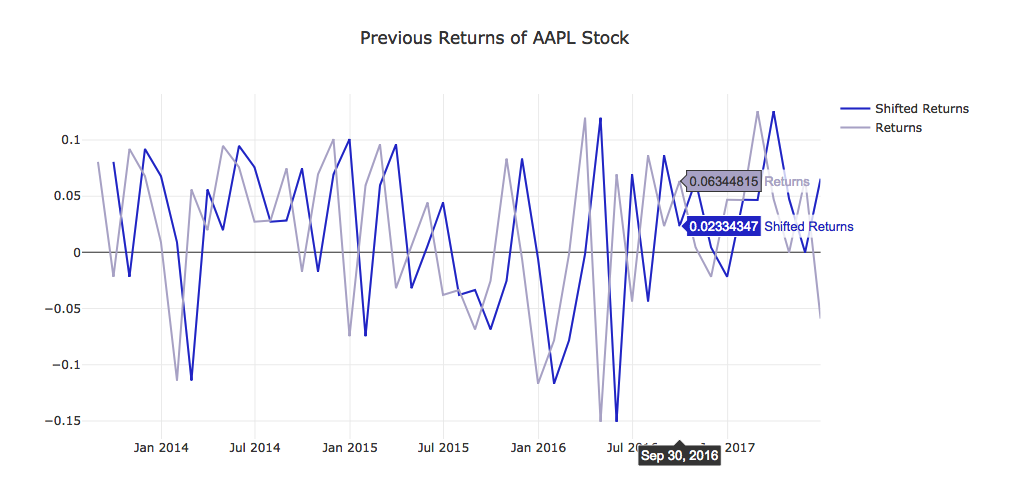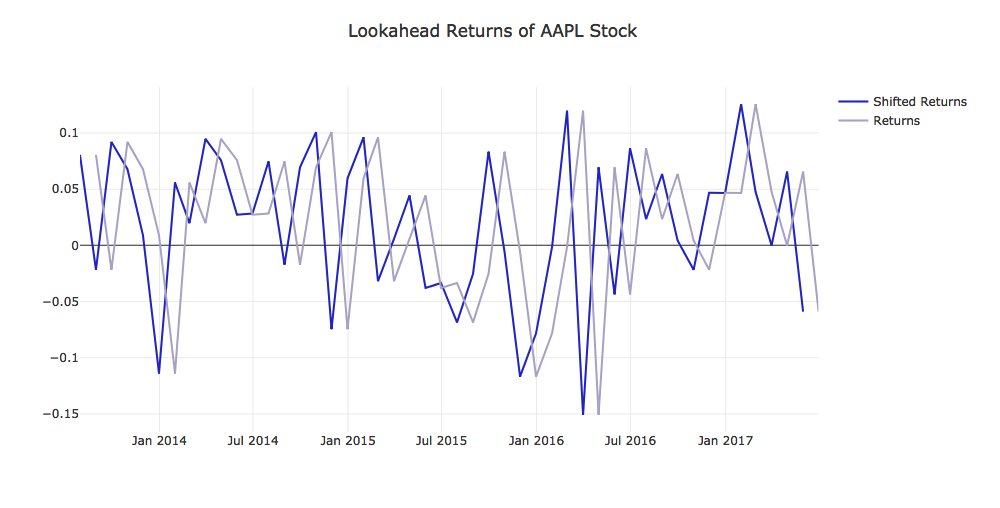A trading signal is a sequence of trading actions, or results that can be used to take trading actions. A common form is to produce a "long" and "short" portfolio of stocks on each date (e.g. end of each month, or whatever frequency you desire to trade at). This signal can be interpreted as rebalancing your portfolio on each of those dates, entering long ("buy") and short ("sell") positions as indicated.

Here's a strategy that we will try:

For each month-end observation period, rank the stocks by previous returns, from the highest to the lowest. Select the top performing stocks for the long portfolio, and the bottom performing stocks for the short portfolio.

Implement the get_top_n function to get the top performing stock for each month. Get the top performing stocks from prev_returns by assigning them a value of 1. For all other stocks, give them a value of 0. For example, using the following prev_returns:

                                     Previous Returns
A         B         C         D         E         F         G
2013-07-08     0.015     0.082     0.096     0.020     0.075     0.043     0.074
2013-07-09     0.037     0.095     0.027     0.063     0.024     0.086     0.025
...            ...       ...       ...       ...       ...       ...       ...

The function get_top_n with top_n set to 3 should return the following:

                                     Previous Returns
A         B         C         D         E         F         G
2013-07-08     0         1         1         0         1         0         0
2013-07-09     0         1         0         1         0         1         0
...            ...       ...       ...       ...       ...       ...       ...

Note: You may have to use Panda's DataFrame.iterrows with Series.nlargest in order to implement the function. This is one of those cases where creating a vecorization solution is too difficult.

def get_top_n(prev_returns, top_n):
"""
Select the top performing stocks

Parameters
----------
prev_returns : DataFrame
Previous shifted returns for each ticker and date
top_n : int
The number of top performing stocks to get

Returns
-------
top_stocks : DataFrame
Top stocks for each ticker and date marked with a 1
"""
# TODO: Implement Function
# use lambda to compute
top_stocks = prev_returns.apply(lambda x: x.nlargest(top_n), axis=1)
# print res
# print(top_stocks) replace na to 0 or 1
top_stocks = top_stocks.applymap(lambda x: 0 if pd.isna(x) else 1)
top_stocks = top_stocks.astype(np.int64)
# print(top_stocks)

project_tests.test_get_top_n(get_top_n)
Tests Passed

View Data

We want to get the best performing and worst performing stocks. To get the best performing stocks, we'll use the get_top_n function. To get the worst performing stocks, we'll also use the get_top_n function. However, we pass in -1*prev_returns instead of just prev_returns. Multiplying by negative one will flip all the positive returns to negative and negative returns to positive. Thus, it will return the worst performing stocks.

top_bottom_n = 50
df_long = get_top_n(prev_returns, top_bottom_n)
df_short = get_top_n(-1*prev_returns, top_bottom_n)
project_helper.print_top(df_long, 'Longed Stocks')
project_helper.print_top(df_short, 'Shorted Stocks')
10 Most Longed Stocks:
INCY, AMD, AVGO, NFX, NFLX, SWKS, ILMN, NVDA, UAL, MU
10 Most Shorted Stocks:
RRC, CHK, FCX, MRO, FTI, DVN, GPS, WYNN, SPLS, MAT

Projected Returns

It's now time to check if your trading signal has the potential to become profitable!

We'll start by computing the net returns this portfolio would return. For simplicity, we'll assume every stock gets an equal dollar amount of investment. This makes it easier to compute a portfolio's returns as the simple arithmetic average of the individual stock returns.

Implement the portfolio_returns function to compute the expected portfolio returns. Using df_long to indicate which stocks to long and df_short to indicate which stocks to short, calculate the returns using lookahead_returns. To help with calculation, we've provided you with n_stocks as the number of stocks we're investing in a single period.

def portfolio_returns(df_long, df_short, lookahead_returns, n_stocks):
"""
Compute expected returns for the portfolio, assuming equal investment in each long/short stock.

Parameters
----------
df_long : DataFrame
Top stocks for each ticker and date marked with a 1
df_short : DataFrame
Bottom stocks for each ticker and date marked with a 1
Lookahead returns for each ticker and date
n_stocks: int
The number number of stocks chosen for each month

Returns
-------
portfolio_returns : DataFrame
Expected portfolio returns for each ticker and date
"""
# TODO: Implement Function
# print()

# print("df_long:")
# print(df_long)
# print()

#print("df_short:")
#print(df_short)
#print()

#print("n_stocks:")
#print(n_stocks)
#print()

res = (lookahead_returns*(df_long - df_short)) / n_stocks

# print res
# print("res:")
# print(res)

return res

project_tests.test_portfolio_returns(portfolio_returns)
Tests Passed

View Data

Time to see how the portfolio did.

expected_portfolio_returns = portfolio_returns(df_long, df_short, lookahead_returns, 2*top_bottom_n)
project_helper.plot_returns(expected_portfolio_returns.T.sum(), 'Portfolio Returns')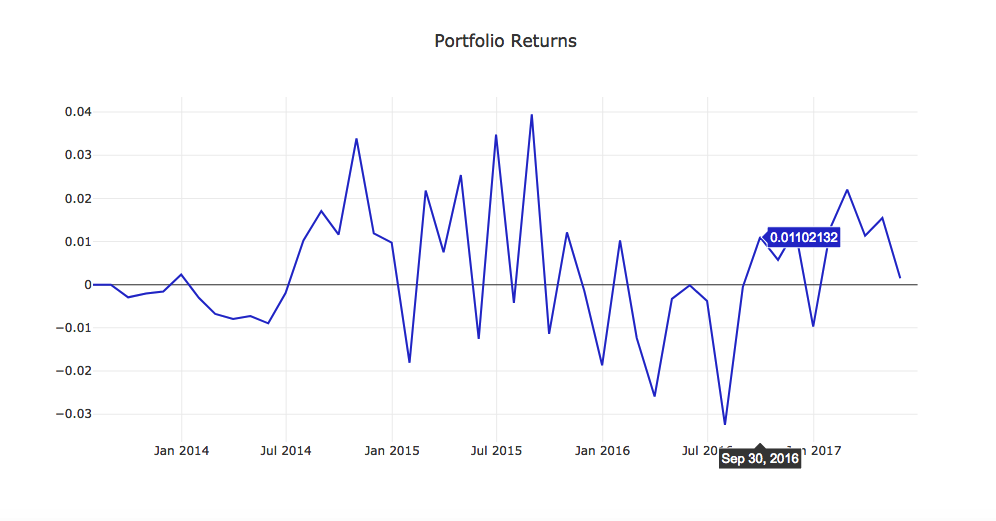Statistical Tests

Annualized Rate of Return

expected_portfolio_returns_by_date = expected_portfolio_returns.T.sum().dropna()
portfolio_ret_mean = expected_portfolio_returns_by_date.mean()
portfolio_ret_ste = expected_portfolio_returns_by_date.sem()
portfolio_ret_annual_rate = (np.exp(portfolio_ret_mean * 12) - 1) * 100

print("""
Mean:                       {:.6f}
Standard Error:             {:.6f}
Annualized Rate of Return:  {:.2f}%
""".format(portfolio_ret_mean, portfolio_ret_ste, portfolio_ret_annual_rate))
Mean:                       0.003076
Standard Error:             0.002180
Annualized Rate of Return:  3.76%

The annualized rate of return allows you to compare the rate of return from this strategy to other quoted rates of return, which are usually quoted on an annual basis.

T-Test

Our null hypothesis ($H_0$) is that the actual mean return from the signal is zero. We'll perform a one-sample, one-sided t-test on the observed mean return, to see if we can reject $H_0$.

We'll need to first compute the t-statistic, and then find its corresponding p-value. The p-value will indicate the probability of observing a t-statistic equally or more extreme than the one we observed if the null hypothesis were true. A small p-value means that the chance of observing the t-statistic we observed under the null hypothesis is small, and thus casts doubt on the null hypothesis. It's good practice to set a desired level of significance or alpha ($\alpha$) before computing the p-value, and then reject the null hypothesis if $p < \alpha$.

For this project, we'll use $\alpha = 0.05$, since it's a common value to use.

Implement the analyze_alpha function to perform a t-test on the sample of portfolio returns. We've imported the scipy.stats module for you to perform the t-test.

Note: scipy.stats.ttest_1samp performs a two-sided test, so divide the p-value by 2 to get 1-sided p-value

from scipy import stats

def analyze_alpha(expected_portfolio_returns_by_date):
"""
Perform a t-test with the null hypothesis being that the expected mean return is zero.

Parameters
----------
expected_portfolio_returns_by_date : Pandas Series
Expected portfolio returns for each date

Returns
-------
t_value
T-statistic from t-test
p_value
Corresponding p-value
"""
# TODO: Implement Function
t_test_results = stats.ttest_1samp(expected_portfolio_returns_by_date, 0)
t_value = t_test_results
p_value = t_test_results / 2

return t_value, p_value

project_tests.test_analyze_alpha(analyze_alpha)
Tests Passed

View Data

Let's see what values we get with our portfolio. After you run this, make sure to answer the question below.

t_value, p_value = analyze_alpha(expected_portfolio_returns_by_date)
print("""
Alpha analysis:
t-value:        {:.3f}
p-value:        {:.6f}
""".format(t_value, p_value))
Alpha analysis:
t-value:        1.411
p-value:        0.082517

Question: What p-value did you observe? And what does that indicate about your signal?

#TODO: Put Answer In this Cell
My p-value is 0.082517, and this is greater than our α of 0.05.

Submission

Now that you're done with the project, it's time to submit it. Click the submit button in the bottom right. One of our reviewers will give you feedback on your project with a pass or not passed grade. You can continue to the next section while you wait for feedback.